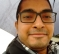## Correct method to add AWGNStarted by 6 years ago8 replieslatest reply 6 years ago239 views

Hi,

I have to mix two signals WiFi and ZigBee. What should be the correct method to add AWGN after chanel effects

A . awgn(WiFi + ZigBee)

OR

B. awgn(WiFi) + awgn(ZigBee)

Additionally, the ZigBee which is 4 MHz wide need to be upsampled to 20 MHz in order to mix with WiFi.

So if option B is correct, the upsampling should be done before or after adding awgn.

Regards

Sumit

[ - ]Option A.

The noise is added at the receiver and should be added according to your sampling bandwidth regardless of signal power. For Eb/N0 you should normalize the values according to signal bandwidth.

[ - ]I could not understand this properly. Can you explain it again please

"For Eb/N0 you should normalize the values according to signal bandwidth"

[ - ]Eb is the energy per bit.  N0 is the noise energy per bit.  White noise comes in power per Hz -- you need to solve for that to get the right noise level.

[ - ]The "A" in AWGN stands for "additive".  So all that your "awgn(x)" function is doing is adding in white Gaussian noise.

So, now you know.  Think about it, and then you tell me if it should be applied once or twice?

[ - ]Post deleted by author

[ - ]Okk.. I just got it ..so

awgn(a) = a + noise

awgn(b) = b + noise

awgn(a) + awgn(b) = a + b + noise + noise = a + b + 2 * noise

:) Thanks !

[ - ]I think you may be embedded too deep in theory-land.

Gaussian noise comes from the environment and from the receiver.  In general there is no significant amount of Gaussian noise that's added by the transmitter.  And it's ADDITIVE.

$$r(t) = n(t)$$, where $$r(t)$$ is the received signal and $$n(t)$$ is noise.

$$r(t) = A_1 s_1(t) + n(t)$$, where $$s_1(t)$$ is the first transmitted signal and $$A_1$$ is the path gain.

$$r(t) = A_2 s_2(t) + A_1 s_1(t) + n(t)$$, where $$s_2(t)$$ is the second transmitted signal and $$A_2$$ is its path gain.

Etc.

[ - ]